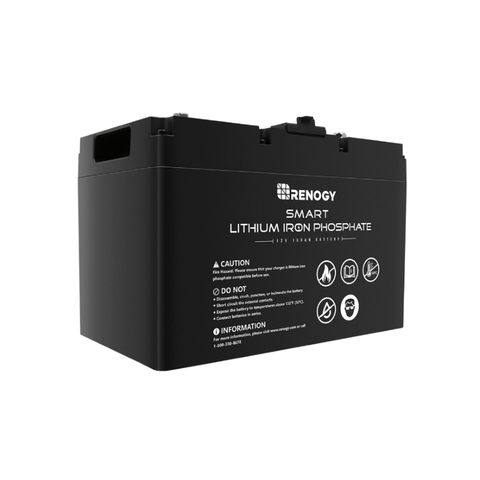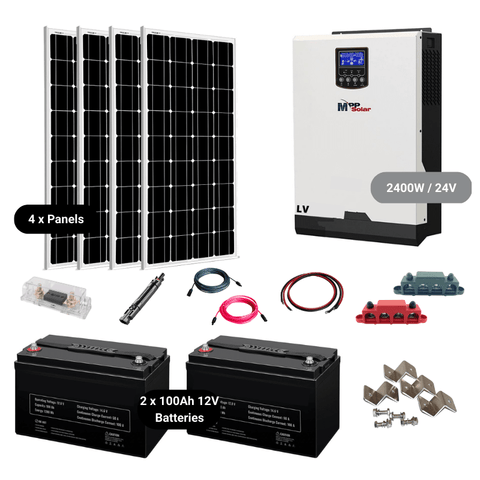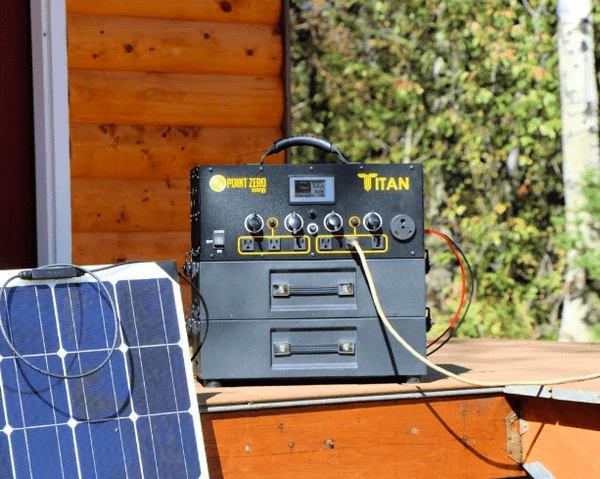Convert Amp Hours to Amps (Electrical Conversion Guide) - ShopSolar.com

# Convert Amp Hours to Amps

The calculations for the conversion from amp hours to amps are often overcomplicated and cause confusion and stress for new solar panel owners. Fortunately, we’re here to demonstrate how to convert amps to watts without having to consult a challenging manual or look at solar forums online.

One of the most practical energy decisions you can make is switching to solar energy and using the sun as your everlasting energy source, especially if you're trying to live a more environmentally friendly lifestyle.

There are several benefits to solar energy, including decreased monthly electricity costs, the potential to make extra money thanks to government tax incentives, and the ability to live completely off the grid.

Deciding to switch is simple, but for solar rookies, comprehending precise solar conversions might be a little intimidating due to the intricate math in solar guides.

Electric current is critical in solar panel systems and having the right conversion metrics is crucial to operating a successful solar system and preventing panel damage.

So, buckle up and put on your solar caps as we talk about how solar panel systems convert amp hours to amps.

## What Is an Ampere or Amp?Circuit terminology abounds in solar electrical guides, but what good are all these overly complex formulas when we don't even understand the difference between amps and amp hours?

Amps, also known as amperes, are the units used to measure how quickly an electric current flow across a circuit. Simply described, they track the number of electrons that flow through a particular spot in a second.

You can use an amp usage calculator to determine the current flowing through a wire at a given time, as well as your daily usage.

## What Are Amp Hours

Unlike an amp which shows the current over a single second, an amp hour refers is the cumulation of one amp for one hour. Ultimately, amp hours can be simply understood by multiplying the amps by the number of hours.

To put it into perspective, let’s say we have a solar panel system that pulls 30 amps, and you use it for 30 minutes. You simply take the 30 amps and multiply it by half an hour to give you the amp hours.

(30 x 0.5)

This gives you a final usage of 15-amp hours (Ah).### What Does This Mean?

The typical Ah rating for solar systems or solar electrical backup systems is usually around 20 hours, which means it takes 20 hours to charge and completely deplete the stored energy.

Solar companies use amp hours (Ah) to express the current produced or consumed by solar panel systems.

Let’s use another example where we use seconds instead of minutes. A portable solar panel that draws 500 amps for 20 seconds uses 500 x 20 / 3,600 (to convert it to hours).

This gives a final amp hour amount of 2.8 Ah.

However, we’re more interested in converting from amp hours to amps, so let us show you how it's done.

### The Conversion

Various calculators and conversion methods are available, including the crucial amp-to-watts calculator, amp-hour calculator, and amp-to-volts calculator. However, in this case, we will be discussing the often-confusing conversion from amp hours to amps.

Now that we have the definitions out of the way, we can finally move on to do the conversion from amp hours to amps.

The conversion uses the same method that we used above to work out the amp hours of an electrical system.The formula that we use is listed as follows:

Amps (A) x Hours (h) = Amp Hours (Ah)

Therefore, if we have the amp hours along with the hours rating for the electrical device, we can easily work out the amps from the amp hours.

For instance, let us say we have an electrical system that has an amp hour rating of 7 Ah and you use the device for 30 minutes.

All you would have to do is substitute the amp hours and hours, which in this case are given in minutes, into the formula above and rearrange. Doing so will give you a new formula that reads as follows.

Amps (A) = Amp Hours (Ah) / Hours (h)

Amps (A) = 7 (Ah) / 0.5 (h)

We get 0.5 hours because we have to convert the 30 minutes into hours by dividing the minutes by 60.

Once it is all into the formula you simply do the math, and you get a final amp rating of 14 A.

That’s how easy it is to work out the amps from amp hours.

Always remember to convert your usage time from seconds or minutes into hours to get the correct amp rating.

## Conclusion

Calculating solar electrical current conversions might be challenging at first, especially if you’re following an intricate guide that uses complicated methods and formulas.

Thankfully, most solar conversion methods rely on a single formula where you can easily substitute factors and get a quick answer.Visit Shop Solar Kits if you need any additional help figuring out an electrical current converter. We provide exceptional customer service and can assist you with any questions regarding solar energy, including system configurations involving solar-powered generators and general solar guidance.From our guide you may learn everything there is to know about solar conversions and how straightforward these calculations are. Solar power is for everyone, and nobody should be scared away from utilizing solar energy because of complicated guides.

Since you are now an expert, you can try your hand at the amps to milliamps conversion.

Let’s play our part in making the environment and atmosphere a cleaner place by switching to solar energy.

Did You Find Our Blog Helpful? Then Consider Checking: